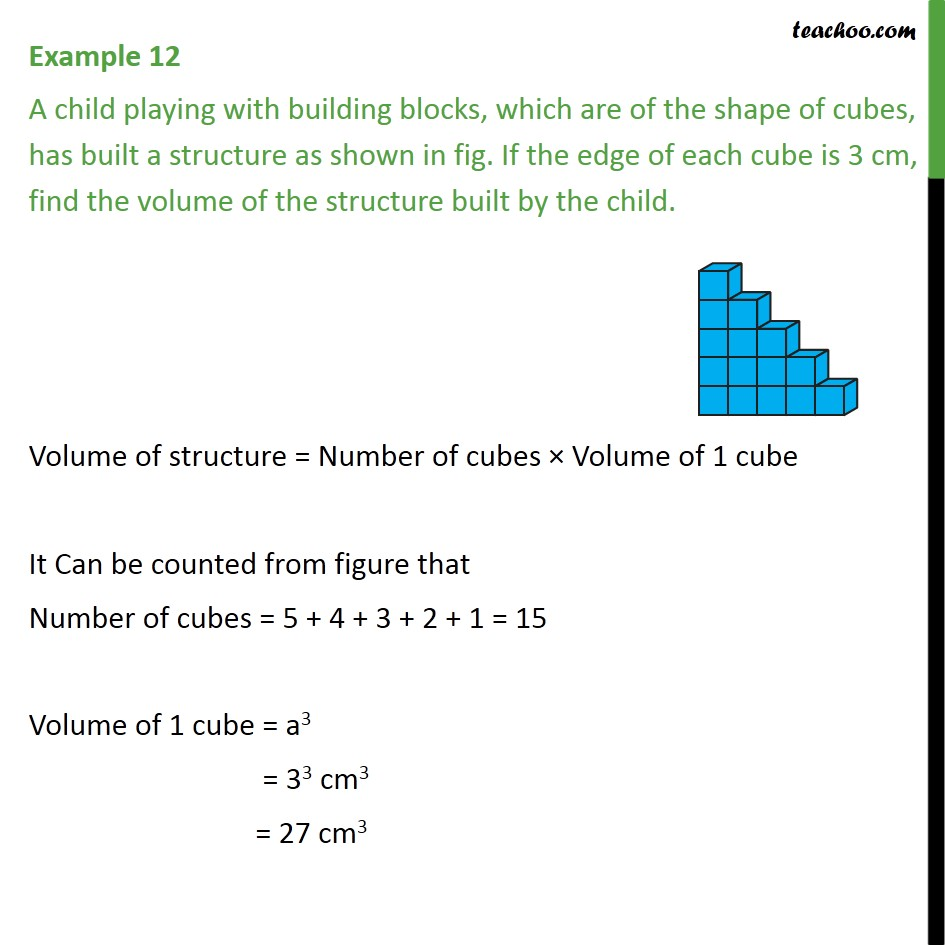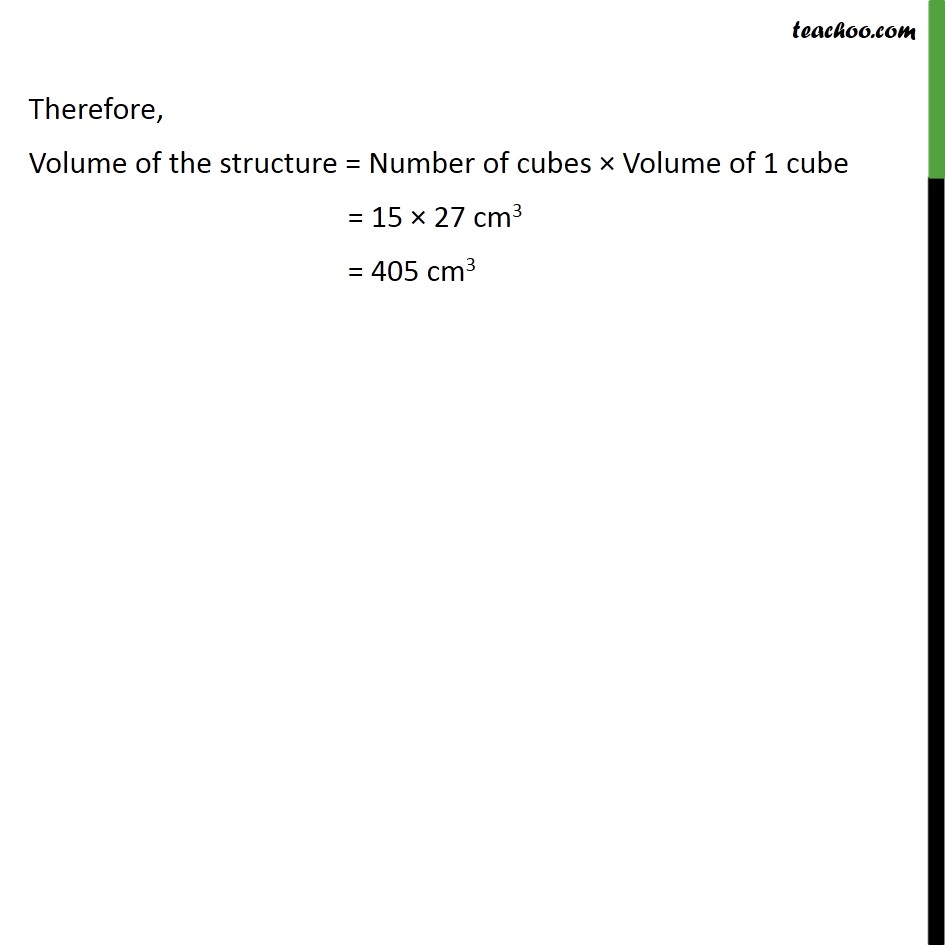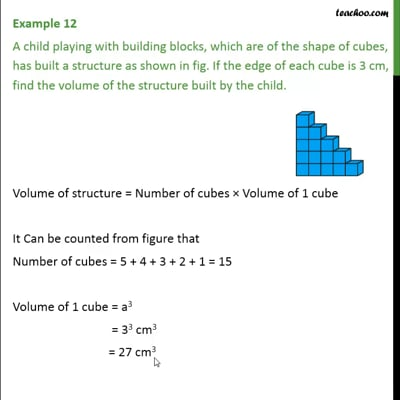Examples

Chapter 13 Class 9 Surface Areas and Volumes
Serial order wiseThis video is only available for Teachoo black users

Introducing your new favourite teacher - Teachoo Black, at only ₹83 per month

### Transcript

Example 12 A child playing with building blocks, which are of the shape of cubes, has built a structure as shown in fig. If the edge of each cube is 3 cm, find the volume of the structure built by the child. Volume of structure = Number of cubes × Volume of 1 cube It Can be counted from figure that Number of cubes = 5 + 4 + 3 + 2 + 1 = 15 Volume of 1 cube = a3 = 33 cm3 = 27 cm3 Therefore, Volume of the structure = Number of cubes × Volume of 1 cube = 15 × 27 cm3 = 405 cm3